# AP Physics C: Mechanics : Calculating Gravitational Forces

## Example Questions

### Example Question #24 : Forces

With what minimum velocity must a rocket be launched from the surface of the moon in order to not fall back down due to the moon's gravity?

The mass of the moon is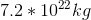and its radius is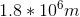.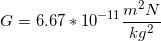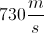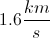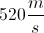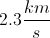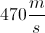Explanation:

Relevant equations: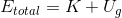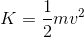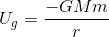For the rocket to escape the moon's gravity, its minimum total energy is zero. If the total energy is zero, the rocket will have zero final velocity when it is infinitely far from the moon. If total energy is less than zero, the rocket will fall back to the moon's surface. If total energy is greater than zero, the rocket will have some final velocity when it is infinitely far away.

For the minimum energy case as the rocket leaves the surface: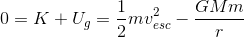Rearrange energy equation to isolate the velocity term.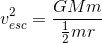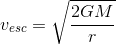Substitute in the given values to solve for the velocity.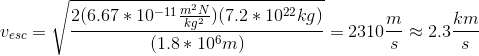### Example Question #25 : Forces

What is the gravitational force of the sun on a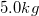book on the earth's surface if the sun's mass is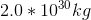and the earth-sun distance is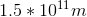?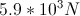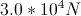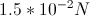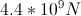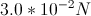Explanation:

Relevant equations: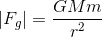Use the given values to solve for the force.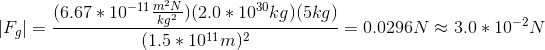### Example Question #26 : Forces

Two spheres of equal mass are isolated in space, and are separated by a distance. If that distance is doubled, by what factor does the gravitational force between the two spheres change?

No changeExplanation:

Newton's law of universal gravitation states: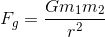We can write two equations for the gravity experienced before and after the doubling: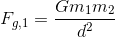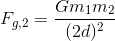The equation for gravity after the doubling can be simplified: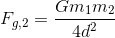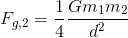Because the masses of the spheres remain the same, as does the universal gravitation constant, we can substitute the definition of Fg1 into that equation: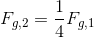The the gravitational force decreases by a factor of 4 when the distance between the two spheres is doubled.

### Example Question #27 : Forces

Two spheres of equal mass are isolated in space. If the mass of one sphere is doubled, by what factor does the gravitational force experienced by the two spheres change?Explanation:

Newton's law of universal gravitation states that:We can write two equations representing the force of gravity before and after the doubling of the mass: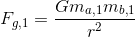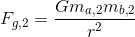The problem gives us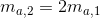and we can assume that all other variables stay constant.

Substituting these defintions into the second equation: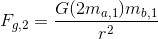This equation simplifies to: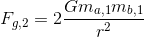Substituting the definition of Fg1, we see: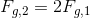Thus the gravitational forces doubles when the mass of one object doubles.

### Example Question #28 : Forces

You are riding in an elevator that is accelerating upwards at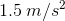, when you note that a block suspended vertically from a spring scale gives a reading of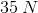.

What does the spring scale read when the elevator is descending at constant speed?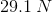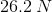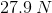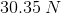Explanation:

When the elevator accelerates upward, we know that an object would appear heavier. The normal force is the sum of all the forces added up, and in this case it is. We know that the normal force has two components, a component from gravity, and a component from the acceleration of the elevator. Using this equation, we can determine the mass of the block, which doesn't change: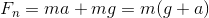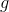is acceleration due to gravity andis the acceleration of the elevator.

When substituting in the values, we get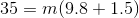Solving for, we get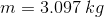Since the elevator is descending at constant speed, no additional force is applied, therefore the force that the spring scale reads is only due to gravity, which is calculated by: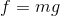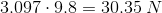### Example Question #29 : Forces

The mass and radius of a planet’s moon are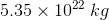and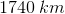respectively.

With what minimum speed would a bullet have to be fired horizontally near the surface of this moon in order for it to never hit the ground?

(Note: You can treat the moon as a smooth sphere, and assume there’s no atmosphere.)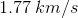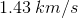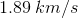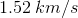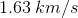Explanation:

To do this problem we have to realize that the force of gravity acting on the bullet is equal to the centripetal force. The equations for gravitational force and centripetal force are as follows: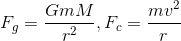If we set the two equations equal to each other, the small(mass of the bullet) will cancel out andwill disappear from the right side of the equation.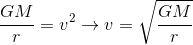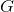is the universal gravitational constant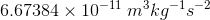is given to be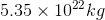andto be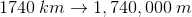.

If we plug everything in, we get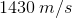or